Showing:

0

77

21d ago

2

3

MIT

# linear-tree

A python library to build Model Trees with Linear Models at the leaves.

linear-tree provides also the implementations of LinearForest and LinearBoost inspired from these works.

## Overview

Linear Trees combine the learning ability of Decision Tree with the predictive and explicative power of Linear Models. Like in tree-based algorithms, the data are split according to simple decision rules. The goodness of slits is evaluated in gain terms fitting Linear Models in the nodes. This implies that the models in the leaves are linear instead of constant approximations like in classical Decision Trees.

Linear Forests generalize the well known Random Forests by combining Linear Models with the same Random Forests. The key idea is to use the strength of Linear Models to improve the nonparametric learning ability of tree-based algorithms. Firstly, a Linear Model is fitted on the whole dataset, then a Random Forest is trained on the same dataset but using the residuals of the previous steps as target. The final predictions are the sum of the raw linear predictions and the residuals modeled by the Random Forest.

Linear Boosting is a two stage learning process. Firstly, a linear model is trained on the initial dataset to obtains predictions. Secondly, the residuals of the previous step are modeled with a decision tree using all the available features. The tree identifies the path leading to highest error (i.e. the worst leaf). The leaf contributing to the error the most is used to generate a new binary feature to be used in the first stage. The iterations continue until a certain stopping criterion is met.

linear-tree is developed to be fully integrable with scikit-learn. `LinearTreeRegressor` and `LinearTreeClassifier` are provided as scikit-learn BaseEstimator to build a decision tree using linear estimators. `LinearForestRegressor` and `LinearForestClassifier` use the RandomForest from sklearn to model residuals. `LinearBoostRegressor` and `LinearBoostClassifier` are available also as TransformerMixin in order to be integrated, in any pipeline, also for automated features engineering. All the models available in sklearn.linear_model can be used as base learner.

## Installation

``````pip install --upgrade linear-tree
``````

The module depends on NumPy, SciPy and Scikit-Learn (>=0.23.0). Python 3.6 or above is supported.

## Usage

##### Linear Tree Regression
``````from sklearn.linear_model import LinearRegression
from lineartree import LinearTreeRegressor
from sklearn.datasets import make_regression
X, y = make_regression(n_samples=100, n_features=4,
n_informative=2, n_targets=1,
random_state=0, shuffle=False)
regr = LinearTreeRegressor(base_estimator=LinearRegression())
regr.fit(X, y)
``````
##### Linear Tree Classification
``````from sklearn.linear_model import RidgeClassifier
from lineartree import LinearTreeClassifier
from sklearn.datasets import make_classification
X, y = make_classification(n_samples=100, n_features=4,
n_informative=2, n_redundant=0,
random_state=0, shuffle=False)
clf = LinearTreeClassifier(base_estimator=RidgeClassifier())
clf.fit(X, y)
``````
##### Linear Forest Regression
``````from sklearn.linear_model import LinearRegression
from lineartree import LinearForestRegressor
from sklearn.datasets import make_regression
X, y = make_regression(n_samples=100, n_features=4,
n_informative=2, n_targets=1,
random_state=0, shuffle=False)
regr = LinearForestRegressor(base_estimator=LinearRegression())
regr.fit(X, y)
``````
##### Linear Forest Classification
``````from sklearn.linear_model import LinearRegression
from lineartree import LinearForestClassifier
from sklearn.datasets import make_classification
X, y = make_classification(n_samples=100, n_features=4,
n_informative=2, n_redundant=0,
random_state=0, shuffle=False)
clf = LinearForestClassifier(base_estimator=LinearRegression())
clf.fit(X, y)
``````
##### Linear Boosting Regression
``````from sklearn.linear_model import LinearRegression
from lineartree import LinearBoostRegressor
from sklearn.datasets import make_regression
X, y = make_regression(n_samples=100, n_features=4,
n_informative=2, n_targets=1,
random_state=0, shuffle=False)
regr = LinearBoostRegressor(base_estimator=LinearRegression())
regr.fit(X, y)
``````
##### Linear Boosting Classification
``````from sklearn.linear_model import RidgeClassifier
from lineartree import LinearBoostClassifier
from sklearn.datasets import make_classification
X, y = make_classification(n_samples=100, n_features=4,
n_informative=2, n_redundant=0,
random_state=0, shuffle=False)
clf = LinearBoostClassifier(base_estimator=RidgeClassifier())
clf.fit(X, y)
``````

More examples in the notebooks folder.

Check the API Reference to see the parameter configurations and the available methods.

## Examples

Show the linear tree learning path: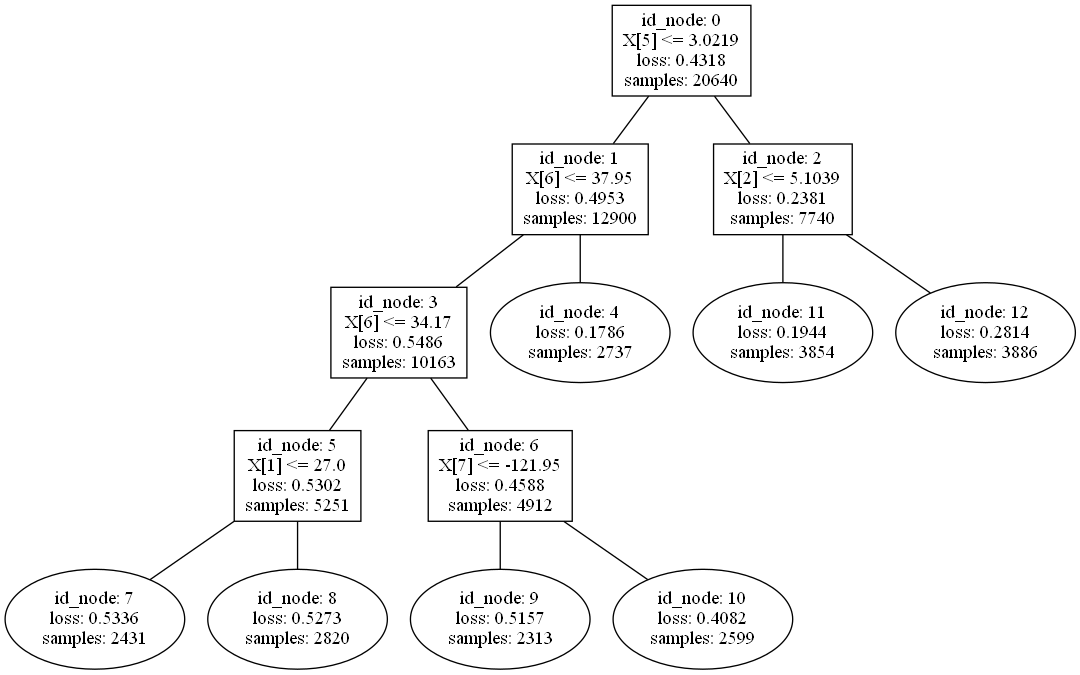Linear Tree Regressor at work: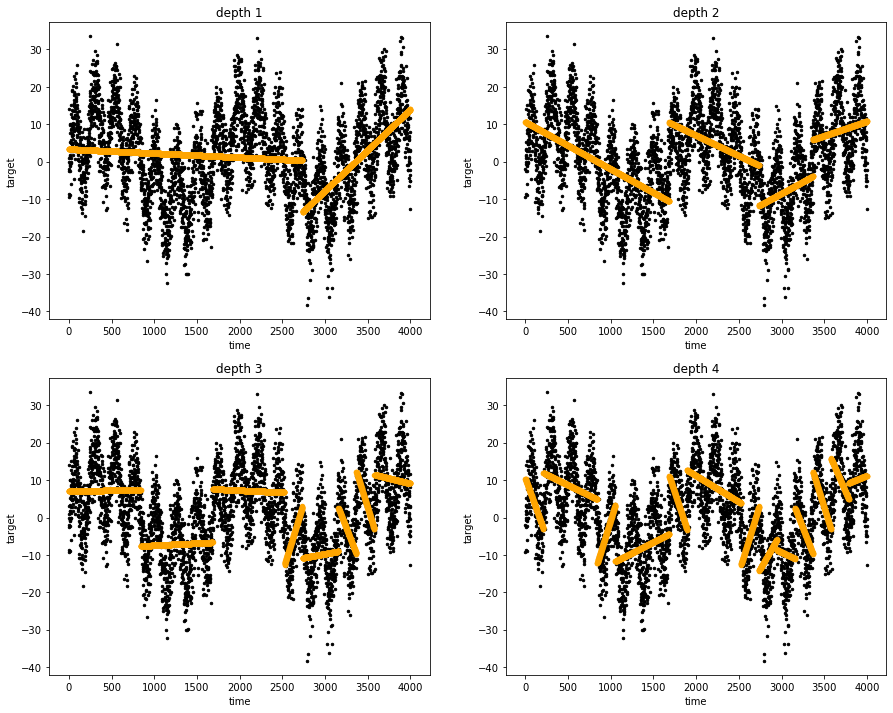Linear Tree Classifier at work: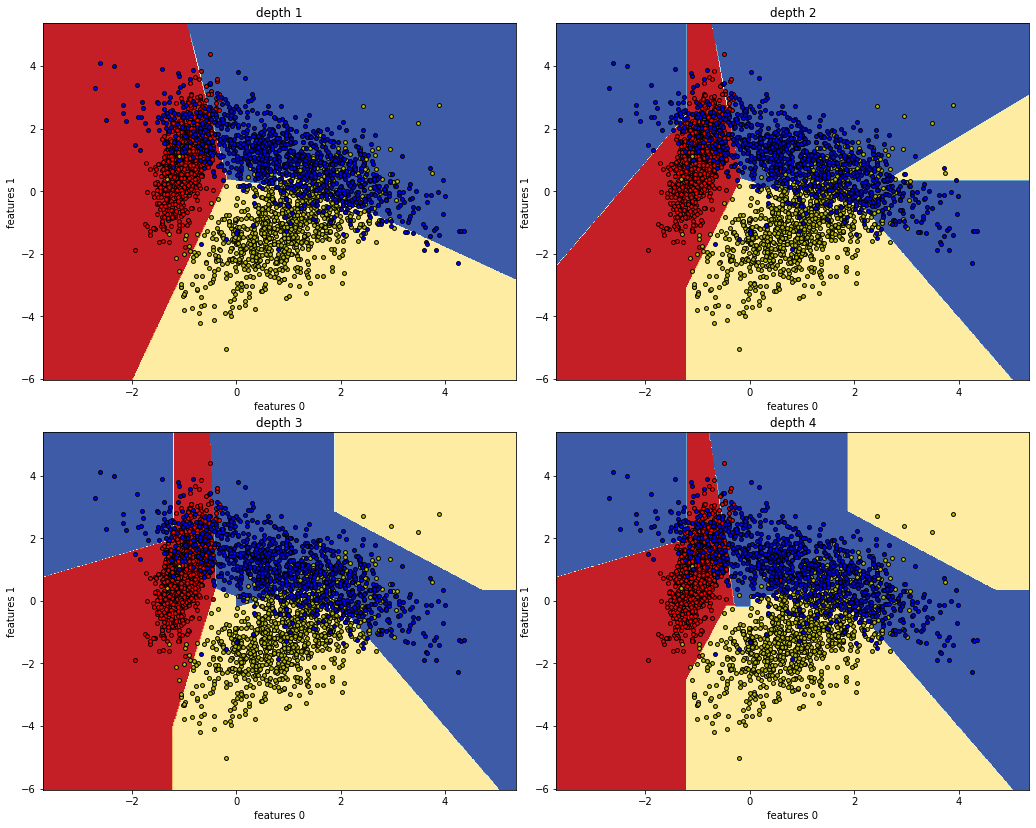Extract and examine coefficients at the leaves: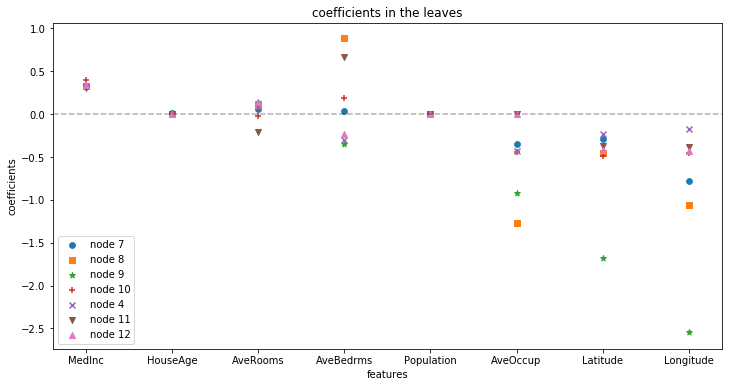Impact of the features automatically generated with Linear Boosting: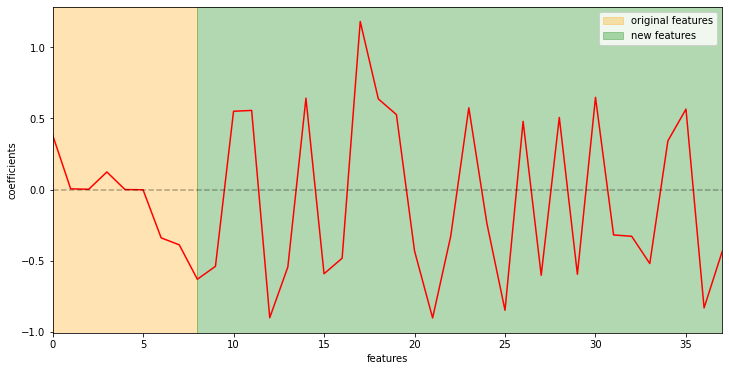Comparing predictions of Linear Forest and Random Forest: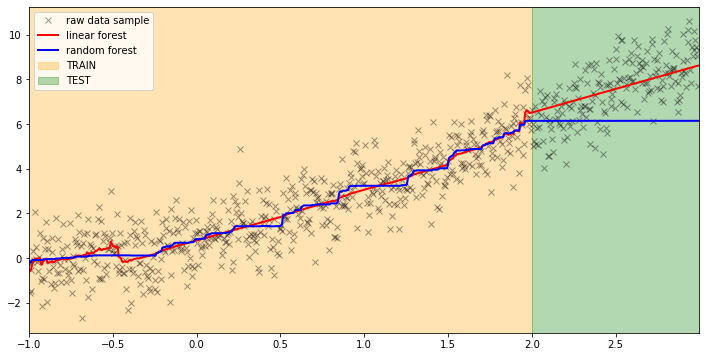• Regression-Enhanced Random Forests. Haozhe Zhang, Dan Nettleton, Zhengyuan Zhu.
• Explainable boosted linear regression for time series forecasting. Igor Ilic, Berk Gorgulu, Mucahit Cevik, Mustafa Gokce Baydogan.

## Rate & Review

Great Documentation0
Easy to Use0
Performant0
Highly Customizable0
Bleeding Edge0
Responsive Maintainers0
Poor Documentation0
Hard to Use0
Slow0
Buggy0
Abandoned0
Unwelcoming Community0
100No reviews found
Be the first to rate

## AlternativesNo alternatives found

## TutorialsNo tutorials found